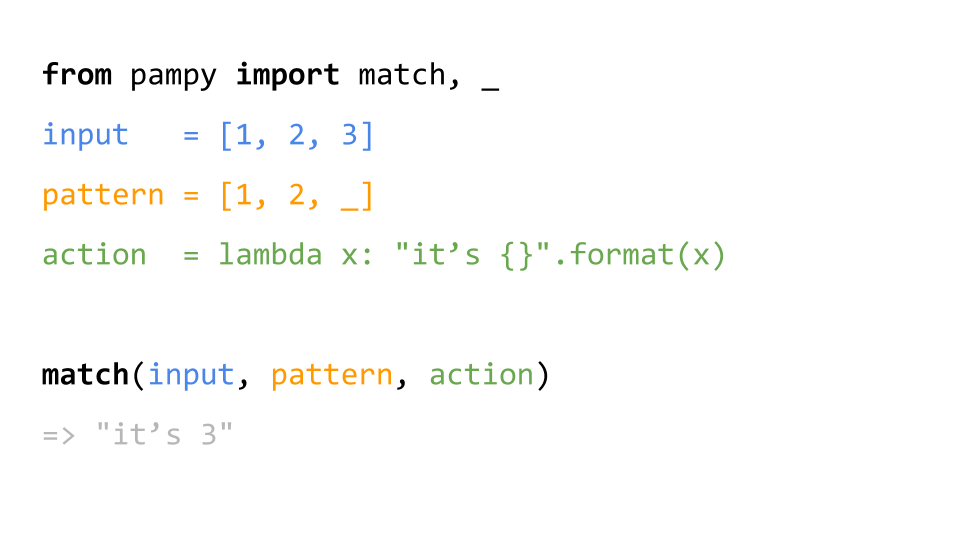signed

QiShunwang

“诚信为本、客户至上”

# 强大的模式匹配工具—Pampy

2021/3/21 10:01:43   来源：

https://github.com/santinic/pampy## 1. 安装

``````\$ pip install pampy
``````

## 2. 使用

HEAD 和 TAIL 能代表某个模式的前面部分或后面部分。

``````from pampy import match, HEAD, TAIL, _
x = [-1, -2, -3, 0, 1, 2, 3]
print(match(x, [-1, TAIL], lambda t: [-1, tuple(t)]))
# => [-1, (-2, -3, 0, 1, 2, 3)]
``````

``````from pampy import match, HEAD, TAIL, _

x = [-1, -2, -3, 0, 1, 2, 3]

print(match(x, [HEAD, _, _, 0, TAIL], lambda h, a, b, t: (set([h, a, b]), tuple(t))))

# => ({-3, -1, -2}, (1, 2, 3))
``````

``````from pampy import match, HEAD, TAIL, _

my_dict = {
'global_setting': [1, 3, 3],
'user_setting': {
'face': ['beautiful', 'ugly'],
'mind': ['smart', 'stupid']
}
}

result = match(my_dict, { _: {'face': _}}, lambda key, son_value: (key, son_value))

print(result)

# => ('user_setting', ['beautiful', 'ugly'])
``````

``````import re

from pampy import match, HEAD, TAIL, _

def what_is(pet):
return match(
pet, re.compile('(\w+)，(\w)\w+鳕鱼\$'), lambda mygod, you: you + "像鳕鱼"
)

print(what_is('我的天，你长得真像鳕鱼'))
# => '你像鳕鱼'``````